# Tense## 30 examples of simple present tense within sentences.

30 examples of simple present tense within sentences. The positive formula for simple present tense is” subject + base form of the verb + s, es, ies + object/complement” Check out the 30 examples of simple present tense. 30 negative examples of simple present tense within sentences. More related Grammars 1000+ Prepositional verbs lists and …## Future perfect Continuous Tense | Definition, formula & exercises

Future perfect Continuous tense Tense which means time, period, or age. In this article, we study about future perfect Continuous tense. Future does not mean present or past. It refers to a period after now means coming time. In order to make it clear we need to put a glance over the meaning of the tense. Future Perfect Continuous Tense Future …## Future Perfect Tense || Definitions, examples & formula

Future Perfect Tense Tense which means time in this article we study about future perfect tense. Future does not mean present or past. It refers to a period after now means coming time. Let’s put glance over the table.  In order to make it clear we need to put a glance over the meaning of …## Future continuous tense | Definition, formula & exercises/worksheet

Future continues tense Tense which means time in this article we study future continuous tense. Future does not mean present or past. It refers to a period after now means coming time. Let’s put a glance over the table. Where I have explained the meaning of the future continuous tense. if you want to learn …## Simple Future Tense | Definition, formula & examples

Simple future tense. Tense which means time in this article we study simple future tense. Future does not mean Present or Past. It refers to a period after now means coming time. Let’s put a glance over the table. Simple Future Tense Simple means not continuous or perfect Future means upcoming time. Time, period, or …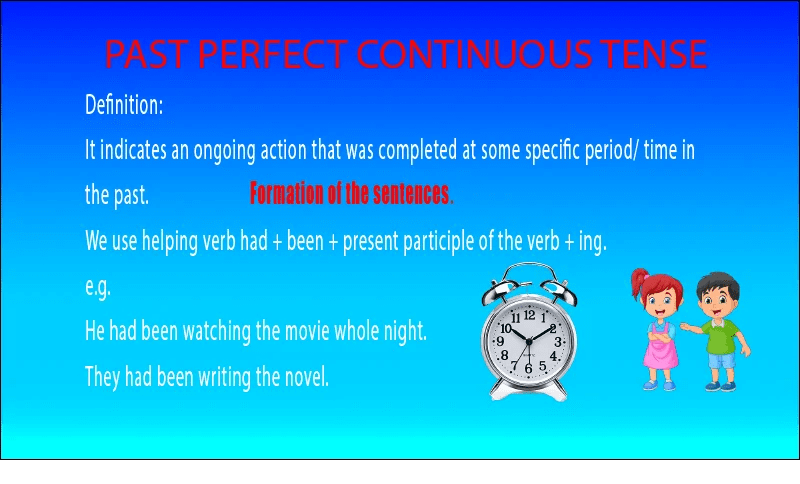## PAST PERFECT CONTINUOUS TENSE( definition, formula, exercises

Past perfect continuous tense Tense which means time in this article we study past perfect continuous tense. It refers to a specific Continuous action or time. Which is quite clear from the meaning.  Let’s put a glance over the table. No: Past Perfect Continuous Tense 1 Before now Past means a time that is not …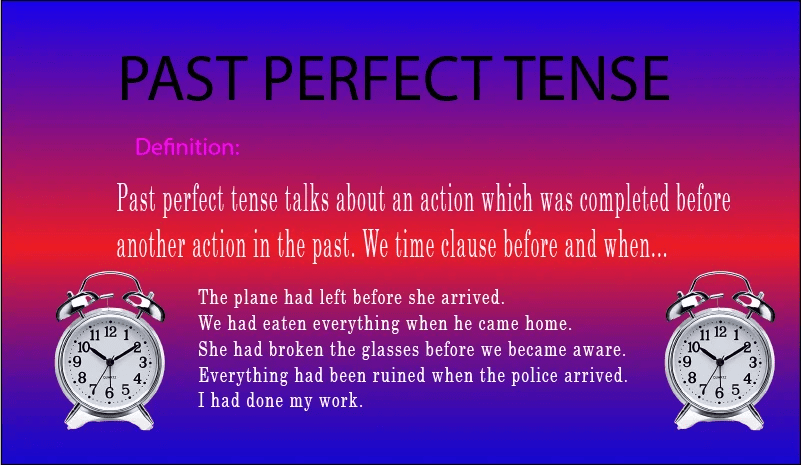## Past Perfect Tense || formula, Examples & exercises/worksheet)

Past Perfect tense Tense which means time in this article we study past perfect tense. It refers to a specific time. Which is quite clear from the meaning.  Past perfect tense talks about an action that is completed before another action in the past. Let’s put a glance over the table where I have explained …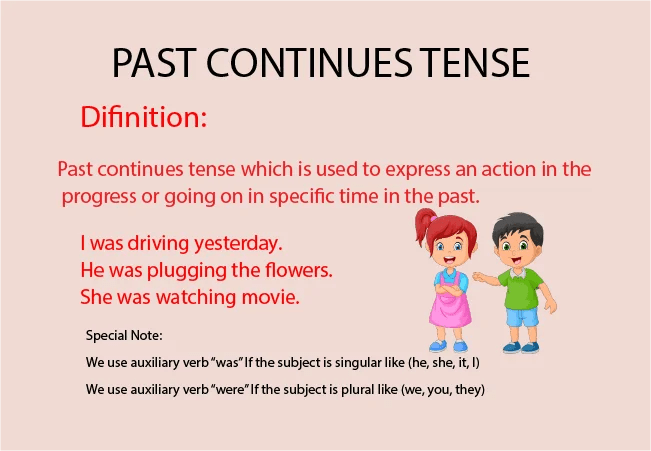## Past continues Tense – definition, formula & exercises/ worksheet

PAST CONTINUOUS TENSE Past continuous tense refers to the past. An action that is going on at the time of speaking. I was writing a letter at 9 pm yesterday. So, a past continuous tense which is really referring to an action going on in the past at a specific time. It is quite different …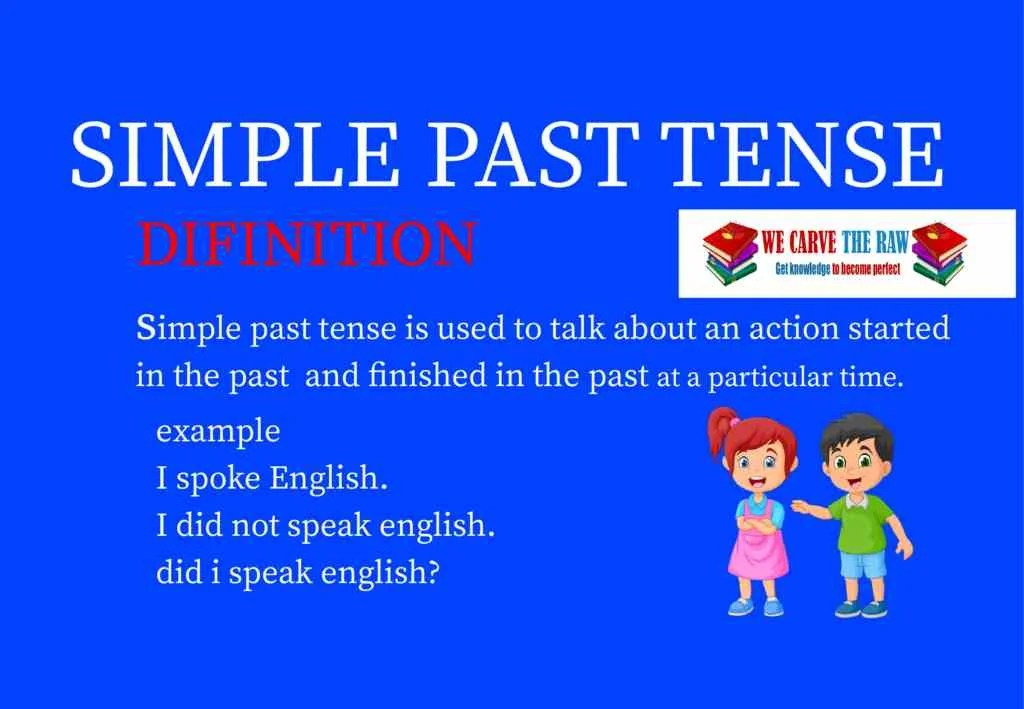## Simple Past Tense | Definition, formula, exercises &worksheet)

Simple Past Tense. Tense is taken from the ‘Latin’ word which means time. In English grammar, we have ‘3’ tenses that talk about three different timings. Like the present, past, and future. Each of these tenses has its 4 kinds. In this article, we study simple past tense that talks about actions that happen in …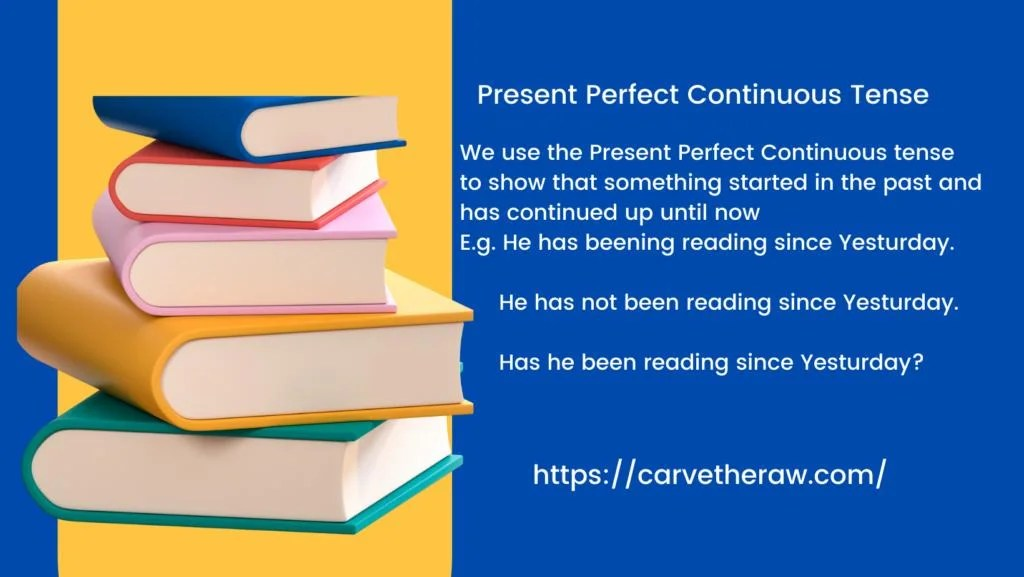## Present Perfect Continuous tense | Definition, formula & exercises/worksheet

Present Perfect Continuous Tense This article will be very interesting because in this article we study tense which is really confusing for some students. Most of the students get amazed when they use the Present Perfect Continuous tense and Present Perfect tense. check out the picture and the definitions given below. Present Perfect Continuous Tesne …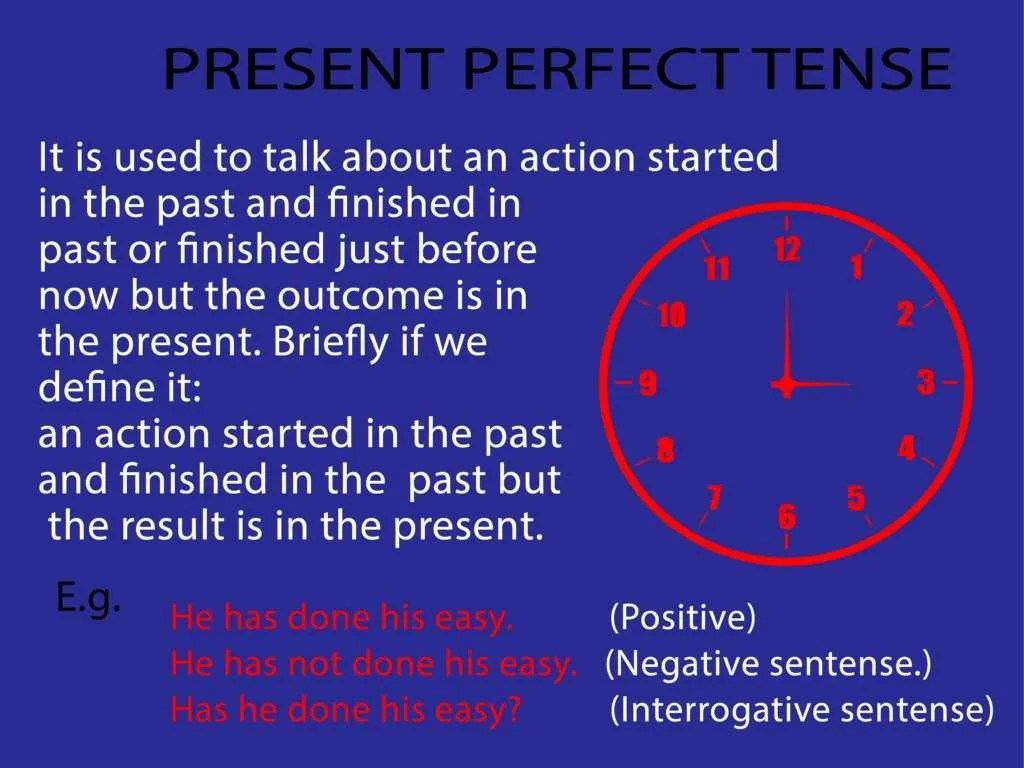## Present Perfect Tense | Since & For Usages

The present perfect tense: Tenses are generally the basic element of the English language which every speaker must know. We have three perfect tenses.  Which are really important. Present Perfect tense, Past perfect tense, and Future perfect tense. They are talking about three different times. We will study the present perfect tense over here. It …## Present Continuous Tense | Definition, Formula & Exercises

Present Continuous Tense. Present Continuous Tense is used to talk about an action that is going on at the time of speaking. In this article, we will study in detail Present Continuous Tense. Tense itself means time. So let’s talk about this continuous tense. We have to grab first the meaning of the title Like …

Scroll to Top